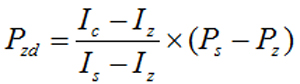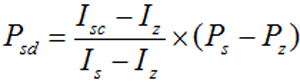Pressure Sensor

Home » FAQ » What to Do for Pressure Sensor Unstable Output Signal

# What to Do for Pressure Sensor Unstable Output Signal

Sometimes output signal of the pressure sensor is unstable, which expressed as the pressure sensor indication to be high or low. Problems with the peripheral accessories of the pressure sensor and the sensor itself will cause this phenomenon. This article will share the methods and techniques for troubleshooting such faults as well as analyze the calibration calculations for pressure sensor zero drift and range upper limit tolerance.
The high or low indication of the pressure sensor means that the pressure sensor output current is out of tolerance. The probability that the pressure sensor itself with some problem causing this fault is extremely low. In most cases, it is caused by the peripheral accessories attached to the sensor are with some malfunctions. For example, in the flow measurement, the balance valve of the three-valve group is not tightly closed or leaked, which is equivalent to indirectly reducing the differential pressure signal entering the differential pressure sensor, resulting in a low output current of the differential pressure sensor. If the pressure guiding tube is blocked or leaked, there will also be a fault that the sensor output current is high or low.
There are some problems in pressure sensor itself will cause the output current to be out of tolerance. One case is that the setting is wrong, which cause the deviation; the other case is the long-term use and environment influence resulting indication out of tolerance.

1. The pressure sensor indication out of tolerance caused by incorrect setting

When the pressure sensor is normal at zero point, the output 0% is consistent with the zero point of the measured pressure or the differential pressure. During the use, if there is a fault that the 0% is inconsistent with the zero point of the measured pressure or the differential pressure, the reason for most of the cases is not that the sensor itself has a problem, but that the settings are incorrect. For example, there is a pressure sensor and its output is 0% in the case of being introduced into the atmosphere, but the displayed pressure value is 0.14MPa. Check it with the hand-held device and found that the auto-zero value is 0.0%; the manual zero value is 1.4%. Use the external zeroing to adjust the pressure display to 0 MPa, and it was found that the output is -0.14%. We know that the value of the output percentage is related to the upper and lower limits of the range. Enter the range setting of the pressure sensor and find that the lower limit of the range is 0.14MPa and the upper limit is 10MPa. The nameplate of the pressure sensor shows that the factory range is 0.14-14MPa. Due to the negligence during the calibration, only the upper limit of the range was modified, and the lower limit of the range was not modified, resulting in the above failure.

2. The pressure sensor indication out of tolerance caused by long term use and the environment influence
• Zero Drift Calibration
The pressure sensor zero drift, that is, the pressure input to the sensor is zero, but the output current is not 4 mA or the display error is outside the allowable range. This problem can usually be solved by clearing with a hand-held device. However, if the zero drift cannot be cleared or the PV value reading error is large after clearing, if there is a standard instrument that meets the requirements, the following method can be used for calibration.
For example, there is a pressure sensor with the range of 0-1 MPa and the accuracy of level 0.2, and its zero output value Ic = 3.9560mA. Clear its zero point by the hand-held device, and the output value is always the same. Calculate the standard pressure value Pzd to be input to the pressure sensor using the following formula.In the formula, Pzd is the pressure at the pressure sensor input end, whose unit is Pa; Ps is the upper limit pressure value of range at the pressure sensor input end, whose unit is Pa; Pz is the zero (lower limit) pressure value of range at the pressure sensor input end, whose unit is Pa; Ic is the pressure sensor output value before calibration, whose unit is mA; Iz is the theoretical output value of the pressure sensor zero point, which is 4mA; Is is the theoretical output value of the upper limit of the pressure sensor range, which is 20mA.
Calculate Pzd=-0.00275MPa according to the formula, so pressurize at the input end of the pressure sensor -0.00275MPa. After the pressure is stable, clear it with the hand-held device and then adjust the input pressure to zero. At this point, the zero output of the pressure sensor is 3.9993 mA, which is within the allowable error range.
• Calibration for the linear out of tolerance
If the pressure sensor linear is out of tolerance within the range, it can be solved by adjusting the upper limit of range. When the input pressure is the upper limit value Ps and the current output upper limit value exceeds the allowable error, modify the upper limit of the pressure sensor to Psd via the Communicator. Psd can be calculated using the following formula. Except for what has been noted, the other symbols in the formula have the same meaning as the above formula.In the formula, Psd is the internally set pressure value after modifying the upper limit of the pressure sensor input, Pa; Isc is the upper limit output value of the pressure sensor before calibration, mA.
It can be seen from formula 2 that the actual upper limit of the pressure sensor is still Ps , that is, the range is constant, but the actual output value is kept consistent with the theoretical value after adjustment to reduce or eliminate the indication error.
For example, there is a pressure sensor with the range of 0-0.4 MPa and the accuracy of level 0.2. When the upper limit pressure value is input, the output current value Isc=19.9643mA. Calculate it according to formula 2, Psd=0.3991MPa. The upper limit of the pressure sensor was changed to 0.3991 MPa with a hand-held device. The input end is applied with an upper limit pressure of 0.4 MPa. At this time, the current output value is 20.0008 mA, which is within the allowable error range, and then calibrate the zero point and the full stroke.
Basically, the above method can solve the zero drift and the upper limit of the range out of tolerance for the pressure sensor. Since these methods are one-point or two-point calibrations, they do not change the pressure sensor range, which only reduce or eliminate the indication error.

I have an Opinion:

Plz Calculate (2 * 8) =
(Your comment will show after approved.)

You may also like: